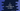# C program to print a two-digit number to word## C program to print a two-digit number to word:

In this post, we will learn how to print a two-digit number to word. It will be a human readable word. For example, if the input number is 11, it will print eleven and if it is 20, it will print twenty.

### Algorithm:

The idea is to break the number to digits.

• We will read the first and the second digits in two different variables.
• Based on the first digit, we can guess the word to print.
• If the first digit is 1, we will read the second digit and based on it, we will print the number in word.
• If the first digit is not 1, we will print the word for that digit e.g. twenty for 2, thirty for 3 etc., and based on the second digit, we will complete the word.

## C program to print two-digit number in word:

Below is the complete program that takes one number as input from the user and print that number in words.

This program will work only for two-digit numbers.

``````#include <stdio.h>

void printIfFirstDigitOne(int secondDigit)
{
if (secondDigit == 0)
{
printf("Ten");
}
else if (secondDigit == 1)
{
printf("Eleven");
}
else if (secondDigit == 2)
{
printf("Twelve");
}
else if (secondDigit == 3)
{
printf("Thirteen");
}
else if (secondDigit == 4)
{
printf("Fourteen");
}
else if (secondDigit == 5)
{
printf("Fifteen");
}
else if (secondDigit == 6)
{
printf("Sixteen");
}
else if (secondDigit == 7)
{
printf("Seventeen");
}
else if (secondDigit == 8)
{
printf("Eighteen");
}
else if (secondDigit == 9)
{
printf("Nineteen");
}
}

void printFirstDigit(int firstDigit)
{
if (firstDigit == 2)
{
printf("Twenty");
}
else if (firstDigit == 3)
{
printf("Thirty");
}
else if (firstDigit == 4)
{
printf("Forty");
}
else if (firstDigit == 5)
{
printf("Fifty");
}
else if (firstDigit == 6)
{
printf("Sixty");
}
else if (firstDigit == 7)
{
printf("Seventy");
}
else if (firstDigit == 8)
{
printf("Eighty");
}
else if (firstDigit == 9)
{
printf("Ninety");
}
}

void printSecondDigit(int secondDigit)
{
if (secondDigit == 1)
{
printf("-one");
}
else if (secondDigit == 2)
{
printf("-two");
}
else if (secondDigit == 3)
{
printf("-three");
}
else if (secondDigit == 4)
{
printf("-four");
}
else if (secondDigit == 5)
{
printf("-five");
}
else if (secondDigit == 6)
{
printf("-six");
}
else if (secondDigit == 7)
{
printf("-seven");
}
else if (secondDigit == 8)
{
printf("-eight");
}
else if (secondDigit == 9)
{
printf("-nine");
}
}

void printInWords(int firstDigit, int secondDigit)
{
if (firstDigit == 1)
{
printIfFirstDigitOne(secondDigit);
}
else
{
printFirstDigit(firstDigit);
printSecondDigit(secondDigit);
}
}

int main()
{
int firstDigit, secondDigit;
printf("Enter the number: ");
scanf("%1d%1d", &firstDigit, &secondDigit);

printf("You have entered: ");
printInWords(firstDigit, secondDigit);

return 0;
}``````

Here,

• firstDigit and secondDigit are two integer variables.
• The user entered number is stored in these variables. The first digit is stored in firstDigit and the second digit is stored in secondDigit.
• printInWords is the main method to print the number in words. We are passing the first and the second digit to this method to print it in words.
• This method checks if the first digit is 1 or not. If it is 1, it calls printIfFirstDigitOne.
• printIfFirstDigitOne takes the second digit and prints the number in words. It can print all numbers from 10 to 19.
• If the first digit is not 1, printInWords will call printFirstDigit and printSecondDigit methods. printFirstDigit method will print the first digit and printSecondDigit will print the second digit.

If you run this program, it will print output as like below:

``````Enter the number: 98
You have entered: Ninety-eight

Enter the number: 11
You have entered: Eleven``````

## C program to print two-digit number in words using switch cases:

The above program uses if-else blocks to find and print the words. We can also use switch cases to do the same thing.

The below program uses switch-case to print two-digit numbers in words:

``````#include <stdio.h>

void printIfFirstDigitOne(int secondDigit)
{
switch (secondDigit)
{
case 0:
printf("Ten");
break;
case 1:
printf("Eleven");
break;
case 2:
printf("Twelve");
break;
case 3:
printf("Thirteen");
break;
case 4:
printf("Fourteen");
break;
case 5:
printf("Fifteen");
break;
case 6:
printf("Sixteen");
break;
case 7:
printf("Seventeen");
break;
case 8:
printf("Eighteen");
break;
case 9:
printf("Nineteen");
break;
}
}

void printFirstDigit(int firstDigit)
{
switch (firstDigit)
{
case 2:
printf("Twenty");
break;
case 3:
printf("Thirty");
break;
case 4:
printf("Forty");
break;
case 5:
printf("Fifty");
break;
case 6:
printf("Sixty");
break;
case 7:
printf("Seventy");
break;
case 8:
printf("Eighty");
break;
case 9:
printf("Ninety");
break;
}
}

void printSecondDigit(int secondDigit)
{
switch (secondDigit)
{
case 1:
printf("-one");
break;
case 2:
printf("-two");
break;
case 3:
printf("-three");
break;
case 4:
printf("-four");
break;
case 5:
printf("-five");
break;
case 6:
printf("-six");
break;
case 7:
printf("-seven");
break;
case 8:
printf("-eight");
break;
case 9:
printf("-nine");
break;
}
}

void printInWords(int firstDigit, int secondDigit)
{
if (firstDigit == 1)
{
printIfFirstDigitOne(secondDigit);
}
else
{
printFirstDigit(firstDigit);
printSecondDigit(secondDigit);
}
}

int main()
{
int firstDigit, secondDigit;
printf("Enter the number: ");
scanf("%1d%1d", &firstDigit, &secondDigit);

printf("You have entered: ");
printInWords(firstDigit, secondDigit);

return 0;
}``````

If you run this program, it will give similar output.